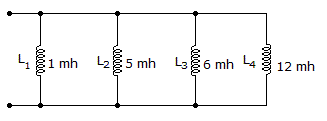# Electronics - Inductors

### Exercise :: Inductors - General Questions

85.

What is the total inductance assuming no mutual inductance?A. 0 mH B. 0.69 mH C. 12 mH D. 24 mH

Answer: Option B

Explanation:

No answer description available for this question. Let us discuss.

86.

A voltage is induced in a transformer secondary winding by the action of the:

 A. secondary magnetic field B. primary turns ratio C. primary magnetic field D. secondary counter emf

Answer: Option C

Explanation:

No answer description available for this question. Let us discuss.

87.

What is electromagnetism?

 A. the magnetic field generated around a conductor when a current passes through it B. the magnetic field generated in a conductor by an external voltage C. the voltage caused by a magnetic field in a conductor D. the current generated by an external magnetic field

Answer: Option A

Explanation:

No answer description available for this question. Let us discuss.

88.

Counter emf or induced voltage is:

 A. inversely proportional to the change in current B. directly proportional to the change in time C. directly proportional to the inductance in henrys D. inversely proportional to the inductance in henrys

Answer: Option C

Explanation:

No answer description available for this question. Let us discuss.

89.

What are the properties that determine the inductance of a coil?

 A. Length of wire, number of turns, type of core material, length of core B. Number of turns, type of wire, length of core, and cross-sectional area of core C. Type of core material, number of turns, cross-sectional area of core, and length of core D. Type of core material, type of wire, number of turns, and cross-sectional area of core

Answer: Option C

Explanation:

No answer description available for this question. Let us discuss.

90.

An inductor is in series with a voltage source, an open switch, and a resistor. The instant the switch is closed, the inductor acts like

 A. a short B. an open C. a resistor D. a voltage source

Answer: Option B

Explanation:

No answer description available for this question. Let us discuss.

91.

What is the phase angle between the source voltage and current, when a 100 mH inductor with a reactance of 6 k, and a 1 kresistor are in series with a source?

 A. 0.1 degrees B. 9.0 degrees C. 61.0 degrees D. 81.0 degrees

Answer: Option D

Explanation:

No answer description available for this question. Let us discuss.

#### Current Affairs 2021

Interview Questions and Answers